#1
0
01. 云栖社区>
2. CDA数据分析师>
3. 博客>
4. 正文

## 做数据分析，Python和R究竟哪个更强？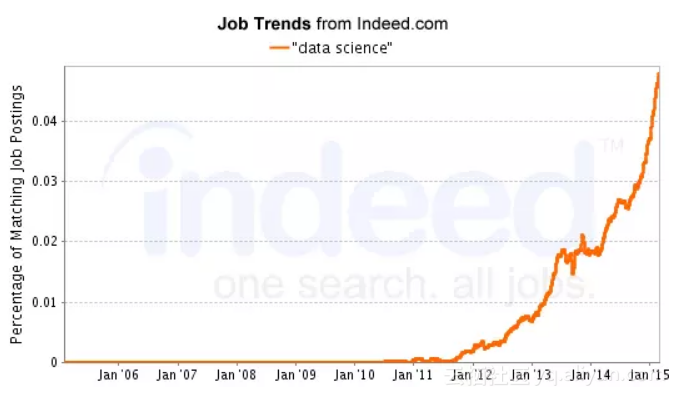1. 简介

Python由Guido van Rossum发明，于1991年首次发布。Python 2.0于2000年发布，8年后Python 3.0发布。Python 3.0有一些主要的语法修正，与Python 2.0不兼容。但是，2to3等Python库可以在两个版本之间自动的转换。Python 2.0计划在2020年停止使用。

R语言由Ross Ihaka和Robert Gentleman于1995年发明。R语言最初是由S语言的一种实现，后者由John Chambers于1976年发明。R语言首个稳定的测试版本1.0.0于2000年发布。目前，由R开发核心团队维护，最新的稳定版本为3.5.1。与Python不同，R在过去没有需要语法转化的重大变化。Guido van Rossum (左) Ross Ihaka (中) Robert Gentleman (右)

Python和R都拥有庞大的用户群体支持。根据Stack Overflow在2017年的调查显示，近45％的数据科学家使用Python作为主要的编程语言。另一方面，11.2％的数据科学家使用R语言。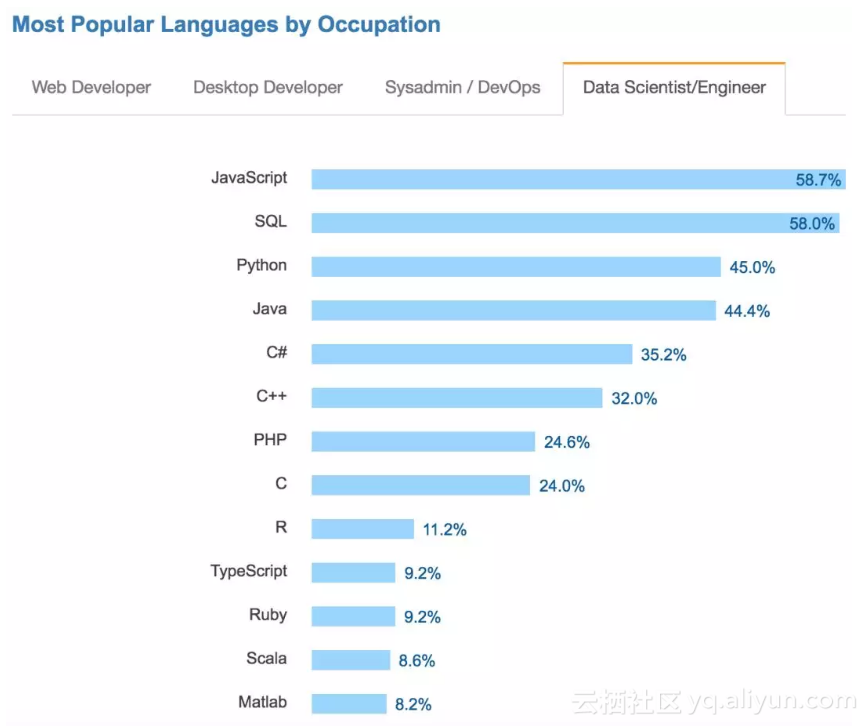2017年开发者调查报告——Stack Overflow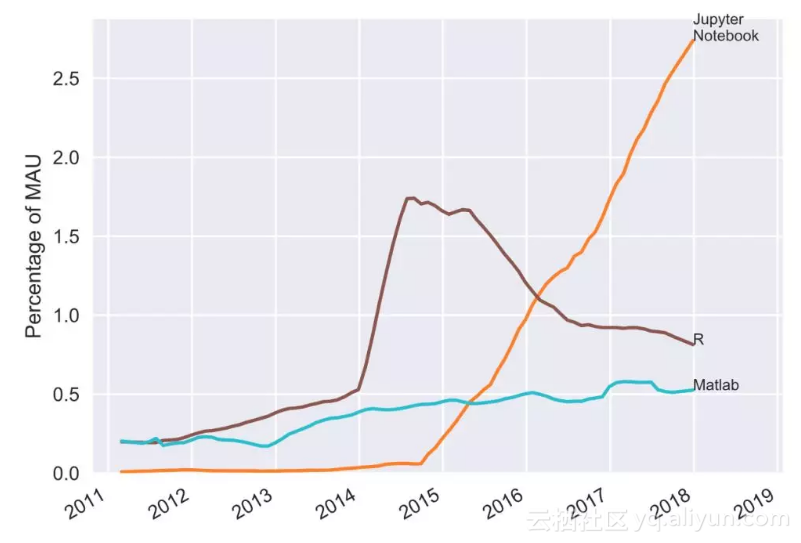GitHub用户对编程语言的排名——Ben Frederickson

2. 可用性

R最初用于研究和学术领域，如今它已不仅仅是一种统计语言。R可以从CRAN(Comprehensive R Archive Network)上轻松下载。CRAN还可用作包管理器，可以下载超过1万多个包。R Studio等流行的开源集成开发环境(IDE)都可以用来运行R语言。

Definitive Guide to Python on Mac OSX

https://medium.com/@briantorresgil/definitive-guide-to-python-on-mac-osx-65acd8d969d0

3. 可视化

ggplot2 教程
http://r-statistics.co/Complete-Ggplot2-Tutorial-Part1-With-R-Code.html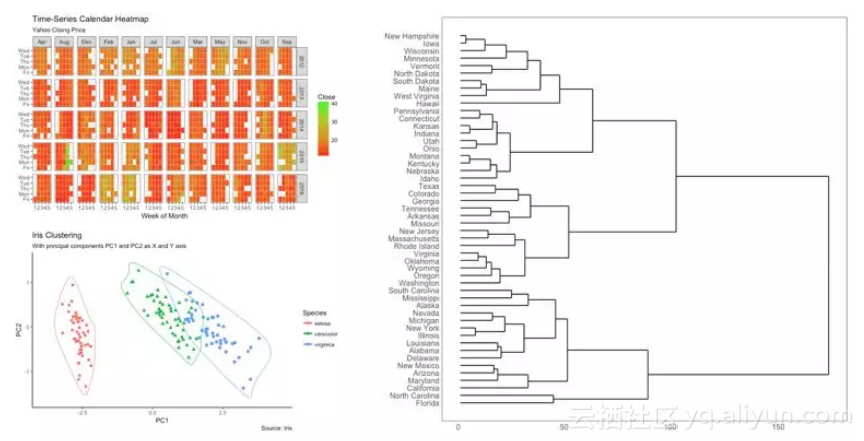ggplot2中的日历热图(左上)、集群图(左下)和层次树图(右下)

Python也有出色的数据可视化库。Matplotlib及其seaborn扩展对可视化和生成统计图很有帮助。我推荐你查看George Seif的相关可视化文章，以便更好地理解Matplotlib。

5 Quick and Easy Data Visualizations in Python with Code
https://towardsdatascience.com/5-quick-and-easy-data-visualizations-in-python-with-code-a2284bae952f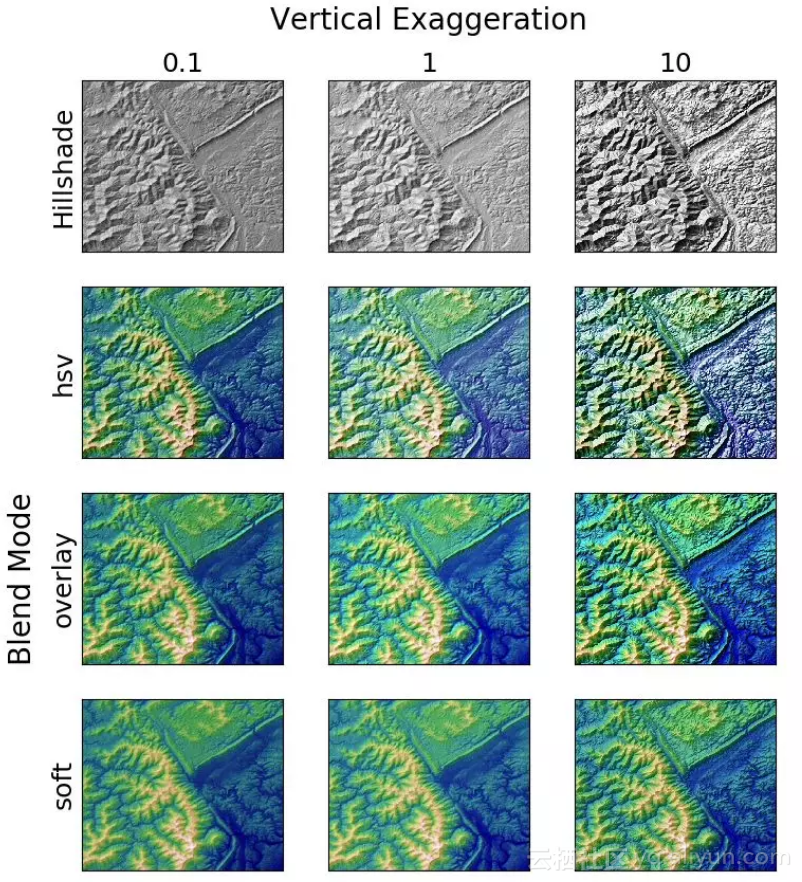Matplotlib的山体阴影效果

Plotly对于Python和R都是很棒的图形库。Plotly(或Plot.ly)是用Python和Django框架构建的。它的前端是用JavaScript构建的，并集成了Python、R、MATLAB、Perl、Julia、Arduino和REST。如果你想构建web应用来展示可视化，我建议你试试Plotly，因为它有带滑块和按钮的交互式图表。

4. 预测分析

Python和R都有强大的预测分析库。在高水平的预测建模中很难比较两者的表现。R语言是专门用作统计语言编写的，因此与Python相比，用R进行搜索与统计建模要更容易。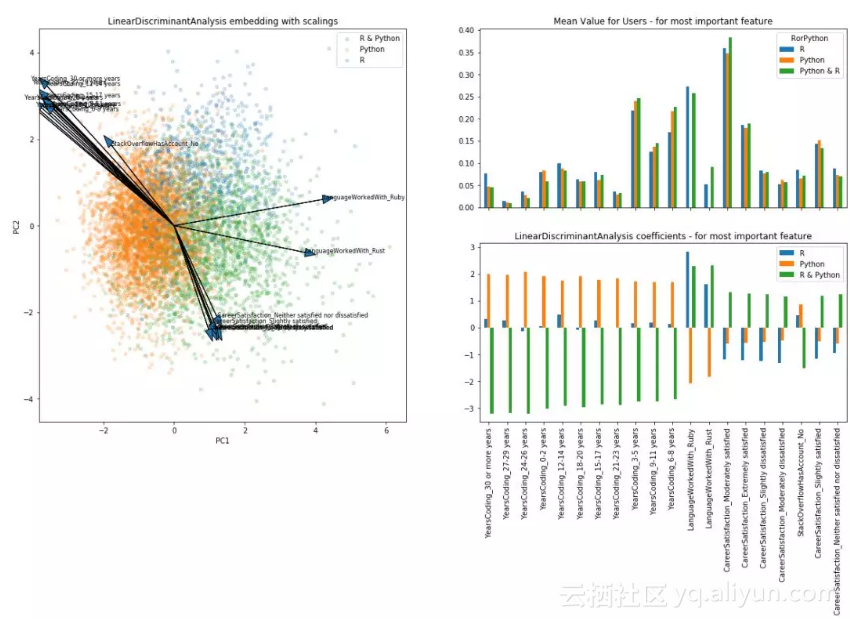``````
``````
`library(datasets)`
``` ```
`#load data`
``` ```
`ir_data<- iris`
``` ```
`head(ir_data)`
``` ```
`#split data`
``` ```
`ir_data<-ir_data[1:100,]`
``` ```
`set.seed(100)`
``` ```
`samp<-sample(1:100,80)`
``` ```
`ir_train<-ir_data[samp,]`
``` ```
`ir_test<-ir_data[-samp,]`
``` ```
`#fit model`
``` ```
`y<-ir_train\$Species; x<-ir_train\$Sepal.Length`
``` ```
`glfit<-glm(y~x, family = 'binomial')`
``` ```
`newdata<- data.frame(x=ir_test\$Sepal.Length)`
``` ```
`#prediction`
``` ```
`predicted_val<-predict(glfit, newdata, type="response")`
``` ```
`prediction<-data.frame(ir_test\$Sepal.Length, ir_test\$Species,predicted_val, ifelse(predicted_val>0.5,'versicolor','setosa'))`
``` ```
`#accuracy`
``` ```
`sum(factor(prediction\$ir_test.Species)==factor(prediction\$ifelse.predicted_val...0.5...versicolor....setosa..))/length(predicted_val)`
``` ```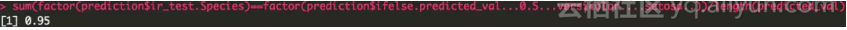R的glm模型准确率达到95%，还不错。
``````
``````
`import pandas as pd`
``` ```
`from sklearn.linear_model import LogisticRegression`
``` ```
`from sklearn.metrics import confusion_matrix`
``` ```
`#load data`
``` ```
`traindf = pd.read_csv("~/data_directory/ir_train")`
``` ```
`testdf = pd.read_csv("~/data_directory/ir_test")`
``` ```
`x = traindf['Sepal.Length'].values.reshape(-1,1)`
``` ```
`y = traindf['Species']`
``` ```
`x_test = testdf['Sepal.Length'].values.reshape(-1,1)`
``` ```
`y_test = testdf['Species']`
``` ```
`#fit model`
``` ```
`classifier = LogisticRegression(random_state=0)`
``` ```
`classifier.fit(x,y)`
``` ```
`#prediction`
``` ```
`y_pred = classifier.predict(x_test)`
``` ```
`#confusion matrix`
``` ```
`confusion_matrix = confusion_matrix(y_test, y_pred)`
``` ```
`print confusion_matrix`
``` ```
`#accuracy`
``` ```
`print classifier.score(x_test, y_test)`
``` ```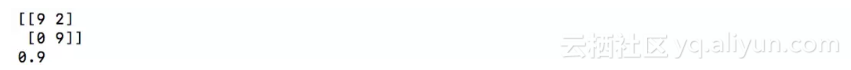Python sklearn的逻辑回归模型准确率达到90%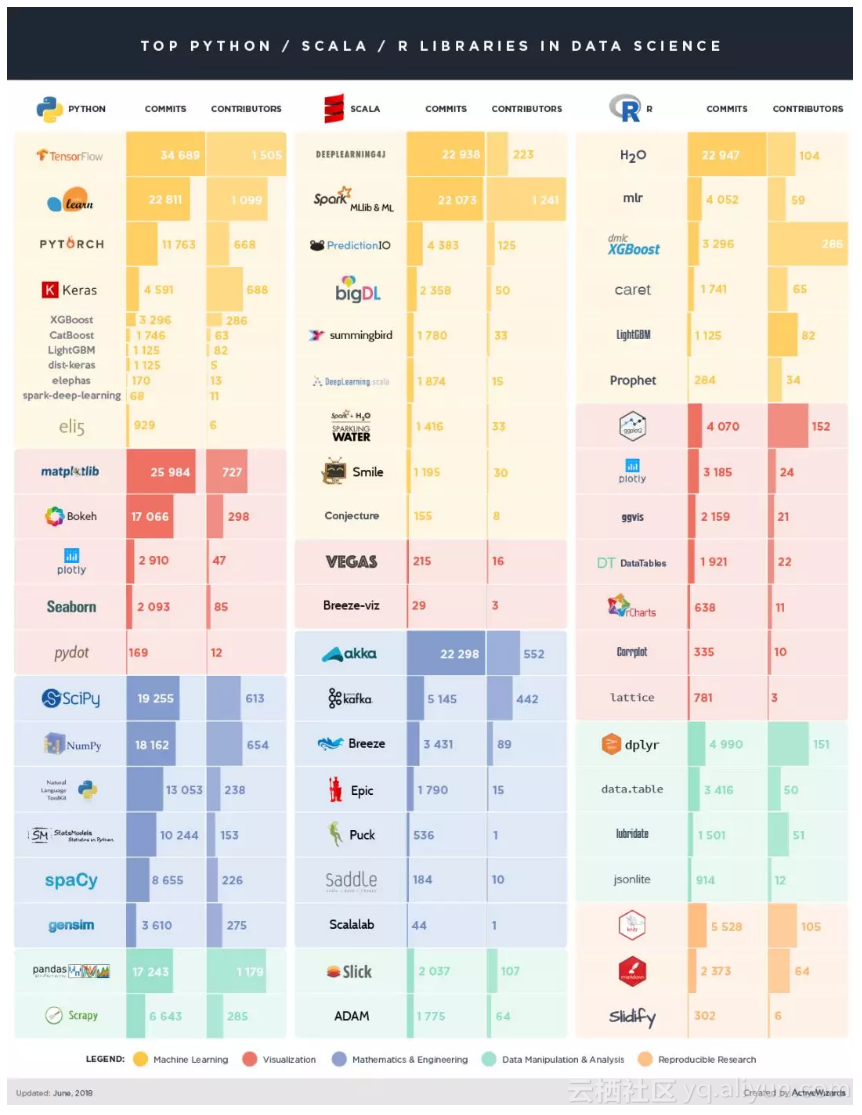5. 性能

R

``````
``````
`require(RJSONIO)`
``` ```
`start_time <- Sys.time()`
``` ```
`json_file <- fromJSON("~/desktop/medium/rpycomparison/yelp-dataset/yelp_academic_dataset_user.json")`
``` ```
`json_file <- lapply(json_file, function(x) {`
``` ```
` x[sapply(x, is.null)] <- NA`
``` ```
` unlist(x)`
``` ```
`})`
``` ```
`df<-as.data.frame(do.call("cbind", json_file))`
``` ```
`end_time <- Sys.time()`
``` ```
`end_time - start_time`
``` ```
`#Time difference of 37.18632 secs`
``` ```

Python

``````
``````
`import time`
``` ```
`import pandas as pd`
``` ```
`start = time.time()`
``` ```
`y1 = pd.read_json('~/desktop/medium/rpycomparison/yelp-dataset/yelp_academic_dataset_user.json', lines = True)`
``` ```
`end = time.time()`
``` ```
`print("Time difference of " + str(end - start) + " seconds"`
``` ```
`#Time difference of 169.13606596 seconds`
``` ```

R加载json文件几乎比Python快5倍。众所周知，Python的加载时间比R快，正如Brian Ray的测试所证明的那样。让我们看看两个程序如何处理大型.csv文件，因为.csv是一种常用的数据格式。我们稍微修改上面的代码来加载 Seattle Library Inventory 数据集，大小约为4.5GB。

Seattle Library Inventory数据集
https://www.kaggle.com/city-of-seattle/seattle-library-collection-inventory/version/15

R

``````
``````
`start_time <- Sys.time()`
``` ```
`df <- read.csv("~/desktop/medium/library-collection-inventory.csv")`
``` ```
`end_time <- Sys.time()`
``` ```
`end_time - start_time`
``` ```
`#Time difference of 3.317888 mins`
``` ```

Python

``````
``````
`import time`
``` ```
`import pandas as pd`
``` ```
`start = time.time()`
``` ```
`y1 = pd.read_csv('~/desktop/medium/library-collection-inventory.csv')`
``` ```
`end = time.time()`
``` ```
`print("Time difference of " + str(end - start) + " seconds")`
``` ```
`#Time difference of 92.6236419678 seconds`
``` ```

R

``````
``````
`#generate data and set boostrap size`
``` ```
`set.seed(999)`
``` ```
`x <- 0:100`
``` ```
`y <- 2*x + rnorm(101, 0, 10)`
``` ```
`n <- 1e5`
``` ```
`#model definition`
``` ```
`fit.mod <- lm(y ~ x)`
``` ```
`errors <- resid(fit.mod)`
``` ```
`yhat <- fitted(fit.mod)`
``` ```
`#bootstrap`
``` ```
`boot <- function(n){`
``` ```
` b1 <- numeric(n)`
``` ```
` b1 <- coef(fit.mod)`
``` ```
` for(i in 2:n){`
``` ```
` resid_boot <- sample(errors, replace=F)`
``` ```
` yboot <- yhat + resid_boot`
``` ```
` model_boot <- lm(yboot ~ x)`
``` ```
` b1[i] <- coef(model_boot)`
``` ```
` }`
``` ```
` return(b1)`
``` ```
`}`
``` ```
`start_time <- Sys.time()`
``` ```
`boot(n)`
``` ```
`end_time <- Sys.time()`
``` ```
`#output time`
``` ```
`end_time - start_time`
``` ```
`#Time difference of 1.116677 mins`
``` ```

Python

``````
``````
`import numpy as np`
``` ```
`import statsmodels.api as sm`
``` ```
`import time`
``` ```
`#generate data and set bootstrap size`
``` ```
`x = np.arange(0, 101)`
``` ```
`y = 2*x + np.random.normal(0, 10, 101)`
``` ```
`n = 100000`
``` ```
`X = sm.add_constant(x, prepend=False)`
``` ```
`#model definition`
``` ```
`fitmod = sm.OLS(y, X)`
``` ```
`results = fitmod.fit()`
``` ```
`resid = results.resid`
``` ```
`yhat = results.fittedvalues`
``` ```
`#bootstrap`
``` ```
`b1 = np.zeros((n))`
``` ```
`b1 = results.params`
``` ```
`start = time.time()`
``` ```
`for i in np.arange(1, 100000):`
``` ```
` resid_boot = np.random.permutation(resid)`
``` ```
` yboot = yhat + resid_boot`
``` ```
` model_boot = sm.OLS(yboot, X)`
``` ```
` resultsboot = model_boot.fit()`
``` ```
` b1[i] = resultsboot.params`
``` ```
`end = time.time()`
``` ```
`#output time`
``` ```
`print("Time difference of " + str(end - start) + " seconds")`
``` ```
`#Time difference of 29.486082077 seconds`
``` ```

R花了几乎两倍的时间来运行bootstrap。鉴于Python通常被视为“慢”编程语言，这是相当令人惊讶的。我开始后悔在完成本科统计学作业时使用R语言而不是Python。

+ 关注